# Optimal control problems for hyperbolic equations with damping terms involving p-Laplacian

## Abstract

In this paper we study optimal control problems for the hyperbolic equations with a damping term involving p-Laplacian. We prove the existence of an optimal control and the Gâteaux differentiability of a solution mapping on control variables. And then we characterize the optimal controls by giving necessary conditions for optimality.

AMS Subject Classification:49K20, 93C20.

## 1 Introduction

In this paper, we are concerned with optimal control problems for the hyperbolic equation with a damping term involving p-Laplacian:

(1.1)

where Ω is a bounded domain in ${\mathbb{R}}^{N}$ with sufficiently smooth boundary Ω, f is a forcing function, and ${y}^{\mathrm{\prime }}=\frac{\partial y}{\partial t}$, ${y}^{\mathrm{\prime }\mathrm{\prime }}=\frac{{\partial }^{2}y}{\partial {t}^{2}}$. The background of these variational problems are in physics, especially in solid mechanics. The precise hypotheses on the above system will be given in the next section.

Recently, much research has been devoted to the study of hemivariational inequalities . The research works have mainly considered the existence of weak solutions for differential inclusions of various forms [2, 3, 5, 6, 9]. In particular, the case where the nonlinear wave equation includes a nonlinear damping term in a bounded domain was proved by many authors for both existence and nonexistence of global solutions . Especially,  showed the existence of global weak solutions for (1.1) and the asymptotic stability of the solution by using the Nakao lemma .

On the other hand, it is interesting to mention that optimal control problems for the equation of Kirchhoff type with a damping term have been studied by Hwang and Nakagiri . We can find some articles about the studies on some kinds of semilinear partial differential equations and on quasilinear partial differential equations; see Ha and Nakagiri , Hwang and Nakagiri . Based on these methods, we intend to study optimal control problems for the hyperbolic hemivariational inequality (1.1) due to the theory of Lions  in which the optimal control problems are surveyed on many types of linear partial differential equations.

Our goal in this paper is to extend the optimal control theory in the framework of Lions  to the hyperbolic equation (1.1) involving p-Laplacian with a damping term. Let H be a Hilbert space and let $\mathcal{U}$ be another Hilbert space of control variables, and B be a bounded linear operator from $\mathcal{U}$ into ${L}^{2}\left(0,T;H\right)$, which is called a controller. We formulate our optimal control problem as follows:

(1.2)

The plan of this paper is as follows. In Section 2, the main results besides notations and assumptions are stated. In Section 3, we show the existence of an optimal control $u\in \mathcal{U}$ which minimizes the quadratic cost function. In Section 4, we characterize the optimal controls by giving necessary conditions for optimality. For this we prove the Gâteaux differentiability of the nonlinear mapping $v\to y\left(v\right)$, which is used to define the associated adjoint system.

## 2 Preliminaries

Throughout this paper we denote

For every $q\in \left(1,\mathrm{\infty }\right)$, we denote ${\parallel \cdot \parallel }_{q}={\parallel \cdot \parallel }_{{L}^{q}\left(\mathrm{\Omega }\right)}$. For brevity, we denote ${\parallel \cdot \parallel }_{2}$ by $\parallel \cdot \parallel$. For a Banach space X, we denote by ${\parallel \cdot \parallel }_{X}$ the norm of X.

Define $A:V\to {V}^{\ast }$ by

where ${V}^{\ast }$ denotes the dual space of V and $〈\cdot ,\cdot 〉$ the dual pairing between V and ${V}^{\ast }$. Then the operator A is bounded, monotone, hemicontinuous (see, e.g., ) and

Now, we formulate the following assumption:

1. (H)

We assume that p is an even natural number satisfying

Definition 2.1 A function $y\left(x,t\right)$ is a weak solution to problem (1.1) if for every $T>0$, y satisfies $y\in {L}^{\mathrm{\infty }}\left(0,T;D\left(\mathrm{△}\right)\right)$, ${y}^{\mathrm{\prime }}\in {L}^{2}\left(0,T;D\left(\mathrm{△}\right)\right)\cap {L}^{\mathrm{\infty }}\left(0,T;V\right)$, ${y}^{\mathrm{\prime }\mathrm{\prime }}\in {L}^{2}\left(0,T;H\right)$. And for any $z\in V$ and $f\in {L}^{2}\left(0,T;H\right)$, the following relations hold:

$\left\{\begin{array}{c}{\int }_{0}^{T}\left\{〈{y}^{\mathrm{\prime }\mathrm{\prime }}\left(t\right),z〉+\left(\mathrm{\nabla }{y}^{\mathrm{\prime }}\left(t\right),\mathrm{\nabla }z\right)+\left({|\mathrm{\nabla }y\left(t\right)|}^{p-2}\mathrm{\nabla }y\left(t\right),\mathrm{\nabla }z\right)\right\}\phantom{\rule{0.2em}{0ex}}dt={\int }_{0}^{T}\left(f,z\right)\phantom{\rule{0.2em}{0ex}}dt,\hfill \\ y\left(0\right)={y}_{0},\phantom{\rule{2em}{0ex}}{y}^{\mathrm{\prime }}\left(0\right)={y}_{1}.\hfill \end{array}$

The following theorem is from Jeong et al. .

Theorem 2.1 Let assumptions (H) be satisfied. Then, for ${y}_{0}\in D\left(\mathrm{△}\right)$, ${y}_{1}\in V$ and $f\in {L}^{2}\left(0,T;H\right)$, problem (1.1) has a weak solution.

For any initial data $\left({y}_{0},{y}_{1}\right)\in D\left(\mathrm{△}\right)×V$, we define the solution space $\mathcal{W}={L}^{\mathrm{\infty }}\left(0,T;V\right)\cap {W}^{1,2}\left(0,T;V\right)\cap {W}^{2,2}\left(0,T;H\right)\cap {W}^{1,\mathrm{\infty }}\left(0,T;V\right)$.

Here we remark that $\mathcal{W}$ is continuously imbedded in $C\left(\left[0,T\right];V\right)\cap {C}^{1}\left(\left[0,T\right];V\right)$, so that we assume that there exists

$|\mathrm{\nabla }y\left(t\right)|
(2.1)

Theorem 2.2 Assume that ${y}_{0}\in D\left(\mathrm{△}\right)$, ${y}_{1}\in V$ and $f\in {L}^{2}\left(0,T;H\right)$. The solution mapping $p=\left({y}_{0},{y}_{1},f\right)\to y\left(p\right)$ of $P\equiv D\left(\mathrm{△}\right)×V×{L}^{2}\left(0,T;H\right)$ into $\mathcal{W}$ is strongly continuous. Further, for each ${p}_{1}=\left({y}_{0}^{1},{y}_{1}^{1},{f}_{1}\right)\in P$ and ${p}_{2}=\left({y}_{0}^{2},{y}_{1}^{2},{f}_{2}\right)\in P$, we have the inequality(2.2)

Proof Due to , we can infer that a weak solution $y\in \mathcal{W}$ under the data condition $p=\left({y}_{0},{y}_{1},f\right)\in D\left(\mathrm{△}\right)×V×{L}^{2}\left(0,T:H\right)$. Based on the above results, we prove the inequality (2.2). For that purpose, we denote ${y}_{1}-{y}_{2}\equiv y\left({p}_{1}\right)-y\left({p}_{2}\right)$ by ψ. Then we can observe from (1.1) that

(2.3)

where

$\begin{array}{rcl}\epsilon \left(\psi \right)& =& \left({|\mathrm{\nabla }{y}_{1}|}^{p-2}-{|\mathrm{\nabla }{y}_{2}|}^{p-2}\right)\mathrm{\nabla }{y}_{2}\\ =& \left(\mathrm{\nabla }\psi ,{|\mathrm{\nabla }{y}_{1}|}^{p-3}+\cdots +{|\mathrm{\nabla }{y}_{2}|}^{p-3}\right)\mathrm{\nabla }{y}_{2}.\end{array}$
(2.4)

Multiplying both sides of (2.3) by ${\psi }^{\mathrm{\prime }}$, we have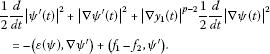(2.5)

And we integrate (2.5) over [0,t] to have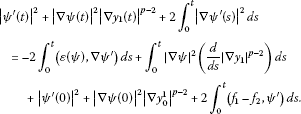(2.6)

The right member of (2.6) can be estimated as follows: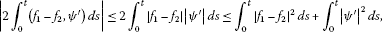(2.7)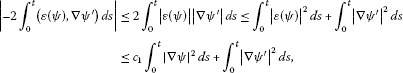(2.8)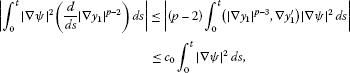(2.9)

where ${c}_{0}$, ${c}_{1}$ are constants. By using the boundedness of (2.1), we obtain (2.8) and (2.9). Finally, we replace the right-hand side of (2.6) by the right members of (2.7)-(2.9) and apply Gronwall’s inequality to the replaced inequality to obtain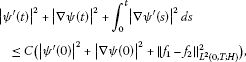(2.10)

where C is a constant. This completes the proof. □

## 3 Existence of an optimal control

Let $\mathcal{U}$ be a Hilbert space of control variables, and let B be a bounded linear operator from $\mathcal{U}$ into ${L}^{2}\left(0,T;H\right)$, which is denoted by

$B\in \mathcal{L}\left(\mathcal{U},{L}^{2}\left(0,T;H\right)\right).$
(3.1)

We consider the following nonlinear control system:

(3.2)

where ${y}_{0}\in D\left(\mathrm{△}\right)$, ${y}_{1}\in V$, and $v\in \mathcal{U}$ is a control. By virtue of Theorem 2.2 and (3.1), we can define uniquely the solution map $v\to y\left(v\right)$ of $\mathcal{U}$ into $\mathcal{W}$. We will call the solution $y\left(v\right)$ of (3.2) the state of the control system (3.2). The observation of the state is assumed to be given by

$z\left(v\right)=Cy\left(v\right),\phantom{\rule{1em}{0ex}}C\in \mathcal{L}\left(\mathcal{W},M\right),$
(3.3)

where C is an operator called the observer and M is a Hilbert space of observation variables. The quadratic cost function associated with the control system (3.2) is given by

(3.4)

where ${z}_{d}\in M$ is a desired value of $y\left(v\right)$ and $R\in \mathcal{L}\left(\mathcal{U},\mathcal{U}\right)$ is symmetric and positive, i.e.,

${\left(Rv,v\right)}_{\mathcal{U}}={\left(v,Rv\right)}_{\mathcal{U}}\ge d{\parallel v\parallel }_{\mathcal{U}}^{2}$
(3.5)

for some $d>0$. Let ${U}_{ad}$ be a closed convex subset of $\mathcal{U}$, which is called an admissible set. An element $u\in {U}_{ad}$ which attains the minimum of $J\left(v\right)$ over ${U}_{ad}$ is called an optimal control for the cost function (3.4).

As indicated in Introduction, we need to show the existence of an optimal control and to give the characterizations of it. The existence of an optimal control u for the cost function (3.4) can be stated by the following theorem.

Theorem 3.1 Assume that the hypotheses of Theorem 2.2 are satisfied. Then there exists at least one optimal control u for the control problem (3.2) with (3.4).

Proof Set $J={inf}_{v\in {U}_{ad}}J\left(v\right)$. Since ${U}_{ad}$ is non-empty, there is a sequence $\left\{{v}_{n}\right\}$ in $\mathcal{U}$ such that

$\underset{v\in {U}_{ad}}{inf}J\left(v\right)=\underset{n\to \mathrm{\infty }}{lim}J\left({v}_{n}\right)=J.$

Obviously, $\left\{J\left({v}_{n}\right)\right\}$ is bounded in ${\mathbb{R}}^{+}$. Then by (3.5) there exists a constant ${K}_{0}>0$ such that

$d{\parallel {v}_{n}\parallel }_{\mathcal{U}}^{2}\le {\left(R{v}_{n},{v}_{n}\right)}_{\mathcal{U}}\le J\left({v}_{n}\right)\le {K}_{0}.$
(3.6)

This shows that $\left\{{v}_{n}\right\}$ is bounded in $\mathcal{U}$. Since ${U}_{ad}$ is closed and convex, we can choose a subsequence (denoted again by $\left\{{v}_{n}\right\}$) of $\left\{{v}_{n}\right\}$ and find a $u\in {U}_{ad}$ such that

(3.7)

as $n\to \mathrm{\infty }$. From now on, each state ${y}_{n}=y\left({v}_{n}\right)\in \mathcal{W}$ corresponding to ${v}_{n}$ is the solution of

(3.8)

By (3.6) the term $B{v}_{n}$ is estimated as

${\parallel B{v}_{n}\parallel }_{{L}^{2}\left(0,T;H\right)}\le {\parallel B\parallel }_{\mathcal{L}\left(\mathcal{U},{L}^{2}\left(0,T;H\right)\right)}{\parallel {v}_{n}\parallel }_{\mathcal{U}}\le {\parallel B\parallel }_{\mathcal{L}\left(\mathcal{U},{L}^{2}\left(0,T;H\right)\right)}\sqrt{{K}_{0}{d}^{-1}}\equiv {K}_{1}.$
(3.9)

Hence it follows from the inequality (2.2), nothing $y\left(\left(0,0,0\right);t\right)\equiv 0$, that

${|{y}_{n}^{\mathrm{\prime }}\left(t\right)|}^{2}+{|\mathrm{\nabla }{y}_{n}\left(t\right)|}^{2}+{\int }_{0}^{t}{|\mathrm{\nabla }{y}_{n}^{\mathrm{\prime }}\left(s\right)|}^{2}\phantom{\rule{0.2em}{0ex}}ds\le C\left({|\mathrm{\nabla }{y}_{0}|}^{2}+{|{y}_{1}|}^{2}+{\parallel f\parallel }_{{L}^{2}\left(0,T;H\right)}^{2}+{K}_{1}^{2}\right)$
(3.10)

for some $C>0$. Combining (3.10) and (3.8), we deduce that

(3.11)

Therefore, by the extraction theorem of Rellich, we can find a subsequence of $\left\{{y}_{n}\right\}$, say again $\left\{{y}_{n}\right\}$, and find a $y\in \mathcal{W}$ such that

(3.12)

By using the fact that $D\left(\mathrm{△}\right)↪V$ is compact and by virtue of (3.11), we can refer to the result of Aubin-Lions Teman’s compact imbedding theorem (cf. Teman ) to verify that $\left\{{y}_{n}\right\}$ is precompact in ${L}^{2}\left(0,T;V\right)$. Hence there exists a subsequence $\left\{{y}_{{n}_{k}}\right\}\subset \left\{{y}_{n}\right\}$ such that

(3.13)

Then we can choose a subsequence of $\left\{{y}_{{n}_{k}}\right\}$, denoted again by $\left\{{y}_{{n}_{k}}\right\}$, such that

(3.14)

Now we will show that

(3.15)

Let $\varphi \left(\cdot \right)\in {L}^{2}\left(0,T;V\right)$ be given. Then we have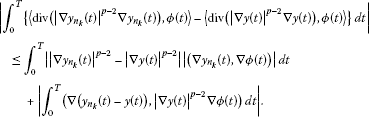(3.16)

Since ${|\mathrm{\nabla }y\left(t\right)|}^{p-2}\mathrm{\nabla }\varphi \in {L}^{2}\left(0,T;H\right)$, it follows from (3.13) and the Hölder inequality that the last term of (3.16) converges to 0 as $k\to \mathrm{\infty }$. By the continuous imbedding $\mathcal{W}↪C\left(\left[0,T\right];V\right)$ and (3.12), we see that the set $\left\{|\mathrm{\nabla }{y}_{{n}_{k}}\left(t\right)|:t\in \left[0,T\right],k=1,2,\dots \right\}$ is bounded with a bound $M>0$. Then, by the Schwartz inequality, the middle term of (3.16) is estimated by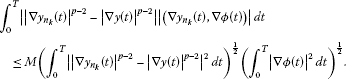(3.17)

We use (3.14) and apply the Lebesgue dominated convergence theorem to have that the term of (3.17) converges to 0 as $k\to \mathrm{\infty }$. This proves (3.15). We replace ${y}_{n}$ by ${y}_{{n}_{k}}$ and take $k\to \mathrm{\infty }$ in (3.8). Since ${y}_{{n}_{k}}\in \mathcal{W}$ are also the weak solutions, as in the standard argument in Dautray and Lions  and Lions and Magenes , we conclude that the limit y is a weak solution of

(3.18)

Also, since equation (3.18) has a unique strong solution $y\in \mathcal{W}$ by Theorem 2.2, we conclude that $y=y\left(v\right)$ in $\mathcal{W}$ by the uniqueness of solutions, which implies $y\left({v}_{n}\right)\to y\left(u\right)$ weakly in $\mathcal{W}$. Since C is continuous on $\mathcal{W}$ and ${\parallel \cdot \parallel }_{M}$ is lower semicontinuous, it follows that

${\parallel Cy\left(u\right)-{z}_{d}\parallel }_{M}\le \underset{n\to \mathrm{\infty }}{lim inf}{\parallel Cy\left({v}_{n}\right)-{z}_{d}\parallel }_{M}.$

It is also clear from ${lim inf}_{n\to \mathrm{\infty }}{\parallel {R}^{\frac{1}{2}}{v}_{n}\parallel }_{\mathcal{U}}\ge {\parallel {R}^{\frac{1}{2}}u\parallel }_{\mathcal{U}}$ that ${lim inf}_{n\to \mathrm{\infty }}{\left(R{v}_{n},{v}_{n}\right)}_{\mathcal{U}}\ge {\left(Ru,u\right)}_{\mathcal{U}}$. Hence

$J=\underset{n\to \mathrm{\infty }}{lim inf}J\left({v}_{n}\right)\ge J\left(u\right).$

But since $J\left(u\right)\ge J$ by definition, we conclude that $J\left(u\right)={inf}_{v\in {U}_{ad}}J\left(v\right)$. This completes the proof. □

## 4 Necessary condition for optimality

In this section we will characterize the optimal controls by giving necessary conditions for optimality. For this it is necessary to write down the necessary optimality condition

(4.1)

and to analyze (4.1) in view of the proper adjoint state system, where $DJ\left(u\right)$ denotes the Gâteaux derivative of $J\left(v\right)$ at $v=u$. That is, we have to prove that the mapping $v\to y\left(v\right)$ of $\mathcal{U}\to \mathcal{W}$ is Gâteaux differentiable at $v=u$. At first we can see the continuity of the mapping. The following lemma follows immediately from Theorem 2.2.

Lemma 4.1 Let $w\in \mathcal{U}$ be arbitrarily fixed. Then

$\underset{\lambda \to 0}{lim}y\left(u+\lambda w\right)=y\left(u\right)\phantom{\rule{1em}{0ex}}\mathit{\text{strongly in}}\mathcal{W}.$

The solution map $v\to y\left(v\right)$ of $\mathcal{U}$ into $\mathcal{W}$ is said to be Gâteaux differentiable at $v=u$ if for any $w\in \mathcal{U}$ there exists a $Dy\left(u\right)\in \mathcal{L}\left(\mathcal{U},\mathcal{W}\right)$ such that

${\parallel \frac{1}{\lambda }\left(y\left(u+\lambda w\right)-y\left(u\right)\right)-Dy\left(u\right)w\parallel }_{\mathcal{W}}\to 0\phantom{\rule{1em}{0ex}}\mathit{\text{as}}\lambda \to 0.$

The operator $Dy\left(u\right)$ denotes the Gâteaux derivative of $y\left(u\right)$ at $v=u$ and the function $Dy\left(u\right)w\in \mathcal{W}$ is called the Gâteaux derivative in the direction $w\in \mathcal{U}$, which plays an important part in the nonlinear optimal control problem.

Theorem 4.1 The map $v\to y\left(v\right)$ of $\mathcal{U}$ into $\mathcal{W}$ is Gâteaux differentiable at $v=u$ and such a Gâteaux derivative of $y\left(v\right)$ at $v=u$ in the direction $v-u\in \mathcal{U}$, say $z=Dy\left(u\right)\left(v-u\right)$, is a unique strong solution of the following problem:

$\left\{\begin{array}{c}{z}^{\mathrm{\prime }\mathrm{\prime }}-\mathrm{△}{z}^{\mathrm{\prime }}-div\left({|\mathrm{\nabla }y\left(u\right)|}^{p-2}\mathrm{\nabla }z\right)=\left(p-2\right){|\mathrm{\nabla }y\left(u\right)|}^{p-3}div\left(\mathrm{\nabla }z\mathrm{\nabla }y\left(u\right)\right)+Bw\hfill \\ \phantom{\rule{1em}{0ex}}\mathit{\text{in}}\mathrm{\Omega }×\left(0,\mathrm{\infty }\right),\hfill \\ z=0\phantom{\rule{1em}{0ex}}\mathit{\text{on}}\partial \mathrm{\Omega }×\left(0,\mathrm{\infty }\right),\hfill \\ z\left(x,0\right)=0,\phantom{\rule{2em}{0ex}}{z}^{\mathrm{\prime }}\left(x,0\right)=0\phantom{\rule{1em}{0ex}}\mathit{\text{in}}\mathrm{\Omega }.\hfill \end{array}$
(4.2)

Proof Let $\lambda \in \left(-1,1\right)$, $\lambda \ne 0$. We set $w=v-u$ and

${z}_{\lambda }={\lambda }^{-1}\left(y\left(u+\lambda w\right)-y\left(u\right)\right).$

Then ${z}_{\lambda }$ satisfies

(4.3)

First we recall the simple equality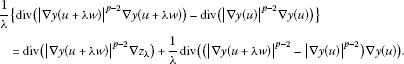Multiplying the weak form of (4.3) by ${z}_{\lambda }^{\mathrm{\prime }}$ and using the above equality, we have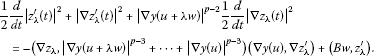(4.4)

We integrate (4.4) over $\left[0,t\right]$ and use the integration by parts to have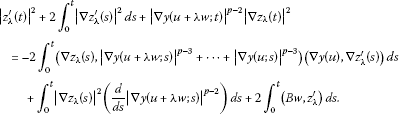(4.5)

By virtue of (2.2) and Young’s inequality, we can estimate each of the integrands of (4.5) as follows: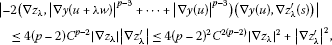(4.6)(4.7)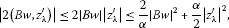(4.8)

where C is a positive constant depending only on the data and $\alpha >0$. Combining (4.5) with (4.6)-(4.8), we have(4.9)

By applying Grownwall’s inequality to (4.9), we obtain

${|{z}_{\lambda }^{\mathrm{\prime }}\left(t\right)|}^{2}+{|\mathrm{\nabla }{z}_{\lambda }\left(t\right)|}^{2}+{\int }_{0}^{t}{|\mathrm{\nabla }{z}_{\lambda }^{\mathrm{\prime }}\left(s\right)|}^{2}\phantom{\rule{0.2em}{0ex}}ds\le {C}_{1}{\parallel Bw\parallel }_{{L}^{2}\left(0,T;H\right)}^{2},$
(4.10)

where ${C}_{1}$ is a constant. The inequality (4.10) provides the boundedness of ${z}_{\lambda }$ and ${z}_{\lambda }^{\mathrm{\prime }}$ in appropriate spaces, and hence via (4.3) we can infer that there exists a $z\in \mathcal{W}$ and a sequence $\left\{{\lambda }_{k}\right\}\subset \left(-1,1\right)$ tending to 0 such that

(4.11)

From Lemma 4.1 and (4.12), we can easily show that

(4.12)

By Lemma 4.1,

(4.13)

so that by (4.12)

This implies that

$\frac{1}{{\lambda }_{k}}\left({|\mathrm{\nabla }y\left(u+{\lambda }_{k}w\right)|}^{p-2}-{|\mathrm{\nabla }y\left(u\right)|}^{p-2}\right)\mathrm{\nabla }y\left(u\right)\to \left(p-2\right)\left(\mathrm{\nabla }{z}_{\lambda },{|\mathrm{\nabla }y\left(u\right)|}^{p-3}\right)\mathrm{\nabla }y\left(u\right).$
(4.14)

Hence we can see from (4.11)-(4.13) that ${z}_{\lambda }\to z=Dy\left(u\right)w$ weakly in $\mathcal{W}$ as $\lambda \to 0$ in which z is a strong solution of (4.2). This convergency can be improved by showing the strong convergence of $\left\{{z}_{\lambda }\right\}$ also in the topology of $\mathcal{W}$.

Subtracting (4.3) from (4.2) and denoting ${z}_{\lambda }-z$ by ${\varphi }_{\lambda }$, we see that

$\left\{\begin{array}{c}{\varphi }_{\lambda }^{\mathrm{\prime }\mathrm{\prime }}-\mathrm{△}{\varphi }_{\lambda }^{\mathrm{\prime }}-div\left({|y\left(u+\lambda w\right)|}^{p-2}\mathrm{\nabla }{\varphi }_{\lambda }\right)=-div\left({\epsilon }_{1}\left({y}_{\lambda }\right)\right)+div\left({\epsilon }_{2}\left({y}_{\lambda },{z}_{\lambda }\right)\right),\hfill \\ {\varphi }_{\lambda }\left(0\right)=0,\phantom{\rule{2em}{0ex}}{\varphi }_{\lambda }^{\mathrm{\prime }}\left(0\right)=0.\hfill \end{array}$
(4.15)

Here in (4.15), for $\lambda \in \left(-1,1\right)$, we set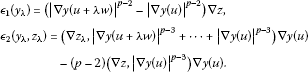From Lemma 4.1 and (4.14), we know that

(4.16)

To estimate ${\varphi }_{\lambda }$, we multiply both sides of (4.15) by ${\varphi }_{\lambda }^{\mathrm{\prime }}$ and integrate it over $\left[0,t\right]$ and use integration by parts to have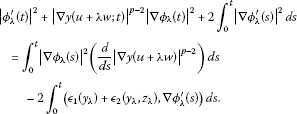(4.17)

The integral parts of the right member of (4.17) can be estimated as follows: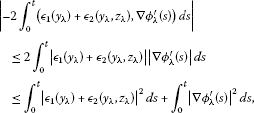(4.18)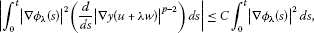(4.19)

where C is a constant. We replace the right-hand side of (4.17) by the right members of (4.18)-(4.19) and we apply Gronwall’s inequality to the replaced inequality, then we arrive at

${|{\varphi }_{\lambda }^{\mathrm{\prime }}\left(t\right)|}^{2}+{|\mathrm{\nabla }{\varphi }_{\lambda }\left(t\right)|}^{2}+{\int }_{0}^{t}{|\mathrm{\nabla }{\varphi }_{\lambda }^{\mathrm{\prime }}\left(s\right)|}^{2}\phantom{\rule{0.2em}{0ex}}ds\le {C}_{2}{\parallel {ϵ}_{1}\left({y}_{\lambda }\right)+{ϵ}_{2}\left({y}_{\lambda },{z}_{\lambda }\right)\parallel }_{{L}^{2}\left(0,T;H\right)}^{2},$
(4.20)

where ${C}_{2}$ is a constant. By virtue (4.16) and (4.20), we deduce that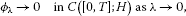(4.21)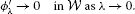(4.22)

Finally, by means of (4.15), (4.21) and (4.22), it follows that

(4.23)

This completes the proof. □

## References

1. Carl S, Heikkila S: Existence results for nonlocal and nonsmooth hemivariational inequalities. J. Inequal. Appl. 2006., 2006: Article ID 79532

2. Miettinen M: A parabolic hemivariational inequality. Nonlinear Anal. 1996, 26: 725–734. 10.1016/0362-546X(94)00312-6

3. Miettinen M, Panagiotopoulos PD: On parabolic hemivariational inequalities and applications. Nonlinear Anal. 1999, 35: 885–915. 10.1016/S0362-546X(97)00720-7

4. Panagiotopoulos PD: Inequality Problems in Mechanics and Applications. Convex and Nonconvex Energy Functions. Birkhäuser, Basel; 1985.

5. Park JY, Kim HM, Park SH: On weak solutions for hyperbolic differential inclusion with discontinuous nonlinearities. Nonlinear Anal. 2003, 55: 103–113. 10.1016/S0362-546X(03)00216-5

6. Park JY, Park SH: On solutions for a hyperbolic system with differential inclusion and memory source term on the boundary. Nonlinear Anal. 2004, 57: 459–472. 10.1016/j.na.2004.02.024

7. Rauch J: Discontinuous semilinear differential equations and multiple valued maps. Proc. Am. Math. Soc. 1977, 64: 277–282. 10.1090/S0002-9939-1977-0442453-6

8. Varga C: Existence and infinitely many solutions for an abstract class of hemivariational inequalities. J. Inequal. Appl. 2005, 2005: 89–105.

9. Nakao M: A difference inequality and its application to nonlinear evolution equations. J. Math. Soc. Jpn. 1978, 30: 747–762. 10.2969/jmsj/03040747

10. Liu L, Wang M: Global existence and blow-up of solutions for some hyperbolic systems with damping and source terms. Nonlinear Anal. 2006, 64: 69–91. 10.1016/j.na.2005.06.009

11. Messaoudi SA: Global existence and nonexistence in a system of Petrovsky. J. Math. Anal. Appl. 2002, 265: 296–308. 10.1006/jmaa.2001.7697

12. Ono K: On global solutions and blow-up solutions of nonlinear Kirchhoff strings with nonlinear dissipation. J. Math. Anal. Appl. 1997, 216: 321–342. 10.1006/jmaa.1997.5697

13. Park JY, Bae JJ: On the existence of solutions of the degenerate wave equations with nonlinear damping terms. J. Korean Math. Soc. 1998, 35: 465–489.

14. Todorova G: Stable and unstable sets for the Cauchy problem for a nonlinear wave equation with nonlinear damping and source terms. J. Math. Anal. Appl. 1999, 239: 213–226. 10.1006/jmaa.1999.6528

15. Jeong JM, Park JY, Park SH: Hyperbolic hemivariational inequalities with boundary source and damping terms. J. Korean Math. Soc. 2009, 24: 85–97. 10.4134/CKMS.2009.24.1.085

16. Hwang J, Nakagiri S: Optimal control problems for Kirchhoff type equation with a damping term. Nonlinear Anal. 2010, 72: 1621–1631. 10.1016/j.na.2009.09.002

17. Ha J, Nakagiri S: Optimal control problems for nonlinear hyperbolic distributed parameter systems with damping terms. Funkc. Ekvacioj 1990, 33: 151–159.

18. Hwang J, Nakagiri S: Optimal control problems for the equation of motion of membrane with strong viscosity. J. Math. Anal. Appl. 2006, 321: 327–342. 10.1016/j.jmaa.2005.07.015

19. Lions JL: Optimal Control of Systems Governed by Partial Differential Equations. Springer, Berlin; 1971.

20. Lions JL: Quelques méthodes de résolution des problémes aux limites non linéaires. Dunod/Gauthier Villars, Paris; 1969.

21. Teman R: Navier Stokes Equation. North-Holland, Amsterdam; 1984.

22. Dautray R, Lions JL: Mathematical analysis and numerical methods for science and technology. 5. In Evolution Problems I. Springer, Berlin; 1992.

23. Lions JL, Magenes E: Non-Homogeneous Boundary Value Problems and Applications. Springer, Berlin; 1972.

## Acknowledgements

This research was supported by the Basic Science Research Program through the National research Foundation of Korea (NRF) funded by the Ministry of Education, Science and Technology (2012-0007560).

## Author information

Authors

### Corresponding author

Correspondence to Jin-Mun Jeong.

### Competing interests

The authors declare that they have no competing interests.

### Authors’ contributions

EYJ carried out the main proof of this manuscript and participated in its design and coordination, JMJ drafted the manuscript and corrected the main theorems.

## Rights and permissions

Reprints and Permissions

Ju, EY., Jeong, JM. Optimal control problems for hyperbolic equations with damping terms involving p-Laplacian. J Inequal Appl 2013, 92 (2013). https://doi.org/10.1186/1029-242X-2013-92

• Accepted:

• Published:

• DOI: https://doi.org/10.1186/1029-242X-2013-92

### Keywords

• optimal control
• hyperbolic equation
• p-Laplacian
• conditions for optimality
• Gâteaux differentiability